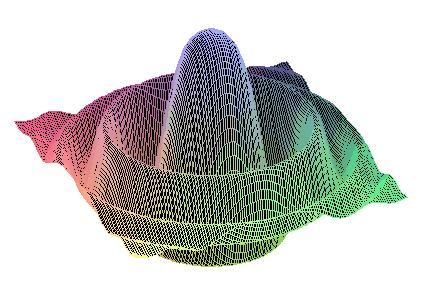DIANA Research Group
DIfferential Algebras and Nonlinear Analysis

Nonlinear Partial Differential Equations

 GF2009    Home    People    Research      Research Topics      Research Projects      START PROJECT      Related Research    Teaching      Current Teaching      Diploma Theses
 Differential algebras of generalized functions provide a framework for unifying and extending existing solution concepts of several important classes of (linear and) nonlinear PDE. An introduction to this line of research can be found in M.Oberguggenberger, Multiplication of distributions and applications to partial differential equations, Pitman Research Notes in Mathematics, Vol 259, Longman, Harlow, 1992. Areas of research Semilinear Hyperbolic Systems Delta Waves Hyperbolic Systems with discontinuous Coefficients Strongly Singular Data Generalized Solutions Quasilinear Hyperbolic Systems Shock Waves in Conservative and Nonconservative Systems Small Viscosity Limits Numerical Methods Special Equations with strongly singular Data Transport Equations Korteweg-de Vries and related Equations Semilinear Parabolic Equations Schrödinger Equations with singular potential Regularity Theory Pseudodifferential Operators on Algebras of Generalized Functions Elliptic Regularity Equations with constant generalized Coefficients
Last changed January 3, 2007 by M.K. and R.S.